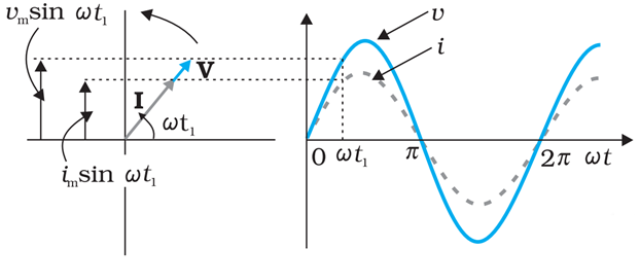### Representation of AC Current and Voltage by Rotating Vectors

The analysis of an ac circuit is by using a phasor diagram. A phasor is a vector which rotates the origin with angular speed ω, as shown in the fig given below.The vertical components of phasors i and v represent the sinusoidally varying quantities v and i. The magnitudes of phasors I and V represent the amplitudes or the peak values im and vm of these oscillating quantities.

The projection of voltage and current phasors on vertical axis,

i.e., im sinωt and vm sinωt respectively represent the value of current and voltage at that instant.